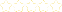• > Jeff Franz
• > Solving One Variable Linear Equations
+# Solving One Variable Linear Equations

Owner: Jeff Franz
##### Description:
Common Core Math 8.EE.7

### Your Quiz Points in this Playlist

Total Possible
0
6 Tutorials in this Playlist

#### Solving One Step Linear Equations with Addition & Subtraction

Author: Jeff Franz
Rating:(4)
This tutorial has a quiz
•• -

#### Solving One Step Linear Equations with Multiplication and Division

Author: Jeff Franz
Rating:(3)
This tutorial has a quiz
•• -

#### Solving Two-Step Linear Equations

Author: Jeff Franz
Rating:(0)
This tutorial has a quiz
•• -

#### Solving Linear Equations with Variables on Both Sides

Author: Jeff Franz
Rating:(0)
This tutorial has a quiz
•• -

#### Solving Multi-Step Linear Equations with Combining Like Terms and Distributing

Author: Jeff Franz
Rating:(1)
This tutorial has a quiz
•• -

#### Solving Multi-Step Linear Equations

Author: Jeff Franz
Rating:(3)
This tutorial has a quiz
•• -